# 第九节、人脸检测之Haar分类器

Haar分类器 = Haar-like特征 + 积分图方法 + AdaBoost +级联；

Haar分类器算法的要点如下：

1. 使用Haar-like特征做检测。
2. 使用积分图（Integral Image）对Haar-like特征求值进行加速。
4. 使用筛选式级联把强分类器级联到一起，提高准确率。

### 一 Haar-like特征

Haar(哈尔)特征分为三类：边缘特征、线性特征、中心特征和对角线特征，组合成特征模板。特征模板内有白色和黑色两种矩形，并定义该模板的特征值为白色矩形像素和减去黑色矩形像素和Haar特征值反映了图像的灰度变化情况。例如：脸部的一些特征能由矩形特征简单的描述，如：眼睛要比脸颊颜色要深，鼻梁两侧比鼻梁颜色要深，嘴巴比周围颜色要深等。但矩形特征只对一些简单的图形结构，如边缘、线段较敏感，所以只能描述特定走向（水平、垂直、对角）的结构。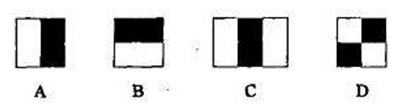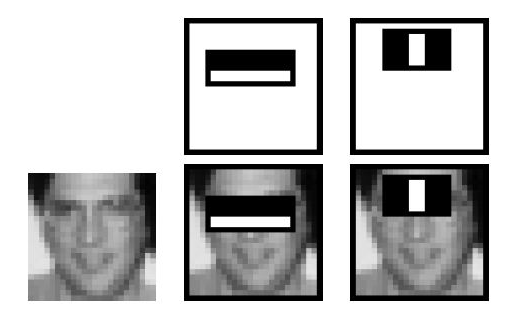### 二、Haar-like特征的计算—积分图

1、用$s(i,j)$表示行方向的累加和，初始化$s(i,-1)=0$；

2、使用$ii(i,j)$表示一个积分图像，初始化$ii(-1,i)$=0；

3、逐行扫描图像，递归计算每个像素$(i,j)$行方向的累加和$s(i,j)$和积分图像$ii(i,j)$的值：

$$s(i,j)=s(i,j-1)+f(i,j)$$

$$ii(i,j)=ii(i-1,j)+s(i,j)$$

4、扫描图像一遍，当到达图像右下角像素时，积分图像$ii$就构建好了。### 三 计算Haar特征值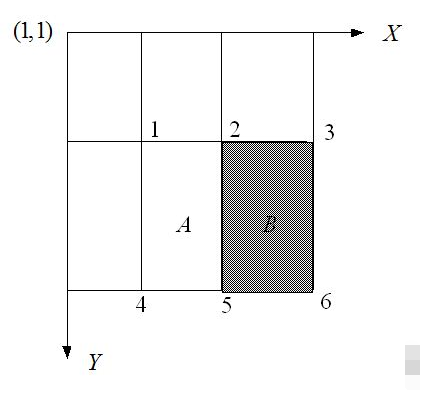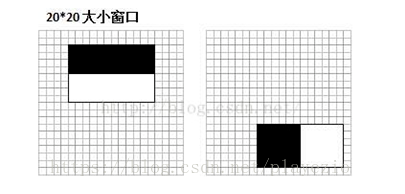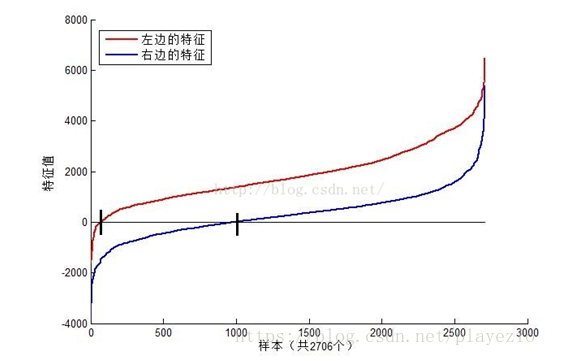（1）在检测窗口通过平移+缩放可以产生一系列Haar特征，这些特征由于位置和大小不同，分类效果也不同；

（2）通过计算Haar特征的特征值，可以有将图像矩阵映射为1维特征值，有效实现了降维。

### 四 Haar特征值归一化(也可以采用标准归一化)

1、计算检测窗口中图像的灰度值和灰度值平方和：

$$sum=\sum i(x,y)$$

$$sq_{sum}=\sum i^2(x,y)$$

2、计算平均值：

$$mean = \frac{sum}{w*h}$$

$$sq_{mean}=\frac{sq_{sum}}{w*h}$$

3、计算归一化因子：

$$varNormFactor=\sqrt{sq_{mean}-mean^2}$$

4、归一化特征值：

$$normValue=\frac{featureValue}{varNormFactor}$$

（2）OpenCV自带的XML分类器中各项参数，如internalNodes和leafValues标签里面的一大堆数字的意义。

#### 1、级联分类器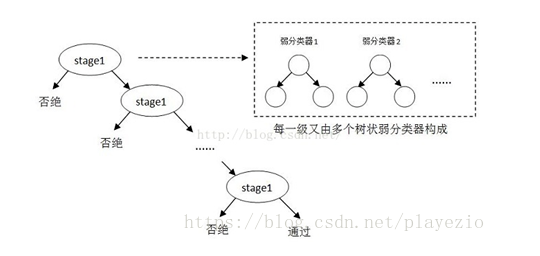#### 2、级联分类器的训练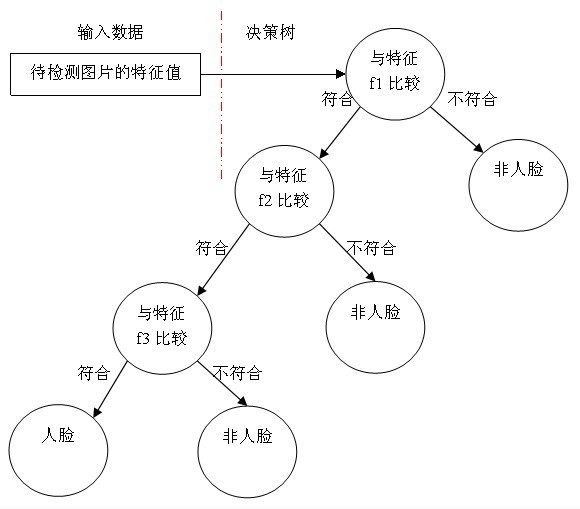1、对于每个特征 $f$，计算所有训练样本的特征值，并将其排序：

2、扫描一遍排好序的特征值，对排好序的表中的每个元素，计算下面四个值：

3、选取当前元素的特征值$F_{k_j}$和它前面的一个特征值$F_{k_{j-1}}$之间的数作为阈值，所得到的弱分类器就在当前元素处把样本分开 —— 也就是说这个阈值对应的弱分类器将当前元素前的所有元素分为人脸（或非人脸），而把当前元素后（含）的所有元素分为非人脸（或人脸）。该阈值的分类误差为：

$$e=min(S^++(T^--S^-),S^-+(T^+-S^+))$$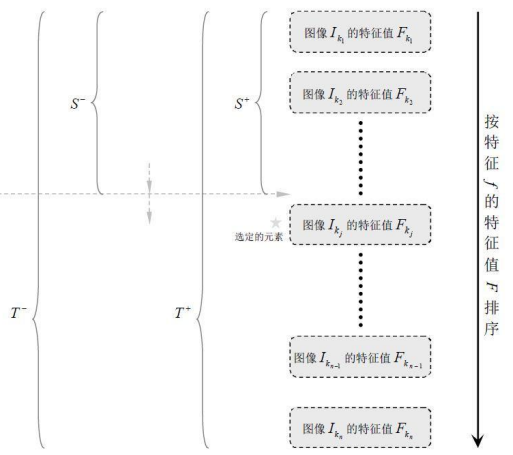1.、给定训练样本集($x_i$,$y_i$)，$i=1,2,3,...N$,共N个样本，$y_i$取值为0(负样本)或者1(正样本)；设人脸正样本的数量为$n_1$，负样本数量为$n_2$； T为训练的最大循环次数；

2.、初始化样本权重为$\frac{1}{n_1+n_2}$，即为训练样本的初始概率分布；

3、$for t=1,...T$:
①权重归一化$$ω_{t,i}=\frac{ω_{t,i}}{\sum\limits_{j-1}^{n}ω_{t,j}}$$

②对每个(种)特征$f_j$，训练一个弱分类器$h_j$（如上），每个分类器只使用一种Haar特征进行训练。分类误差为：$$ε_j=\sum\limits_{i}ω_i|h_j(x_i)-y_i|$$
③从②确定的弱分类器中，找出一个具有最小分类误差的弱分类器$h_t$;
④更新每个样本对应的权重:4、最终形成的强分类器组成为：#### 3、级联分类器的检测

• 一种是不改变搜索窗口的大小，而不断缩放图片，这种方法显然需要对每个缩放后的图片进行区域特征值的运算，效率不高；
• 另一种方法，不断扩大搜索窗口，进行搜索，解决了第一种方法的弱势。

#### 4、总结

1、准备人脸、非人脸样本集；

2、计算特征值和积分图；

3、筛选出T个优秀的特征值（即最优弱分类器）；

5、级联，也就是强分类器的强强联手。

#### 5、XML文件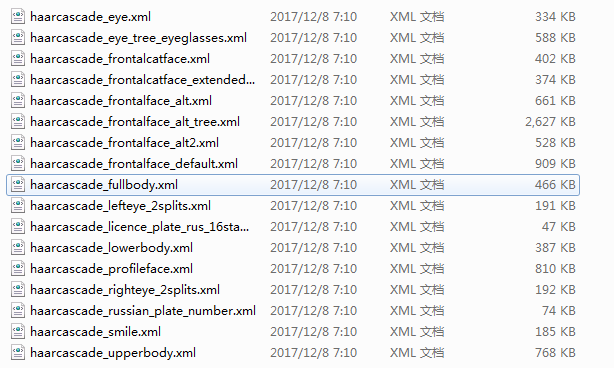### 六 人脸检测

#### 1、静态图像中的人脸检测

# -*- coding: utf-8 -*-
"""
Created on Thu Aug 16 10:32:55 2018

@author: lenovo
"""

'''

'''
import cv2
import numpy as np

#1.静态图像中的人脸检测
def StaticDetect(filename):
#创建一个级联分类器 加载一个 .xml 分类器文件. 它既可以是Haar特征也可以是LBP特征的分类器.

#加载图像
#转换为灰度图
gray_img = cv2.cvtColor(img,cv2.COLOR_BGR2GRAY)
#进行人脸检测，传入scaleFactor，minNegihbors，分别表示人脸检测过程中每次迭代时图像的压缩率以及
#每个人脸矩形保留近似数目的最小值
#返回人脸矩形数组
for (x,y,w,h) in faces:
#在原图像上绘制矩形
img = cv2.rectangle(img,(x,y),(x+w,y+h),(255,0,0),2)
cv2.namedWindow('Face Detected！')
cv2.imshow('Face Detected！',img)
cv2.waitKey(0)
cv2.destroyAllWindows()

#2、视频中的人脸检测
def DynamicDetect():
'''
打开摄像头，读取帧，检测帧中的人脸，扫描检测到的人脸中的眼睛，对人脸绘制蓝色的矩形框，对人眼绘制绿色的矩形框
'''
#创建一个级联分类器 加载一个 .xml 分类器文件. 它既可以是Haar特征也可以是LBP特征的分类器.

#打开摄像头
camera = cv2.VideoCapture(0)
cv2.namedWindow('Dynamic')

while(True):
#读取一帧图像
#判断图片读取成功？
if ret:
gray_img = cv2.cvtColor(frame,cv2.COLOR_BGR2GRAY)
#人脸检测
for (x,y,w,h) in faces:
#在原图像上绘制矩形
cv2.rectangle(frame,(x,y),(x+w,y+h),(255,0,0),2)
roi_gray = gray_img[y:y+h,x:x+w]
#眼睛检测
for (ex,ey,ew,eh) in eyes:
cv2.rectangle(frame,(ex+x,ey+y),(x+ex+ew,y+ey+eh),(0,255,0),2)

cv2.imshow('Dynamic',frame)
#如果按下q键则退出
if cv2.waitKey(100) & 0xff == ord('q') :
break

camera.release()
cv2.destroyAllWindows()

if __name__=='__main__':
#filename = './image/img23.jpg'
#StaticDetect(filename)
DynamicDetect()

    #创建一个级联分类器 加载一个 .xml 分类器文件. 它既可以是Haar特征也可以是LBP特征的分类器.
face_cascade = cv2.CascadeClassifier('./haarcascades/haarcascade_frontalface_default.xml')

    #加载图像
#转换为灰度图
gray_img = cv2.cvtColor(img,cv2.COLOR_BGR2GRAY)

    #进行人脸检测，传入scaleFactor，minNegihbors，分别表示人脸检测过程中每次迭代时图像的压缩率以及
#每个人脸矩形保留近似数目的最小值
#返回人脸矩形数组
for (x,y,w,h) in faces:
#在原图像上绘制矩形
img = cv2.rectangle(img,(x,y),(x+w,y+h),(255,0,0),2)
cv2.namedWindow('Face Detected！')
cv2.imshow('Face Detected！',img)
cv2.waitKey(0)
cv2.destroyAllWindows()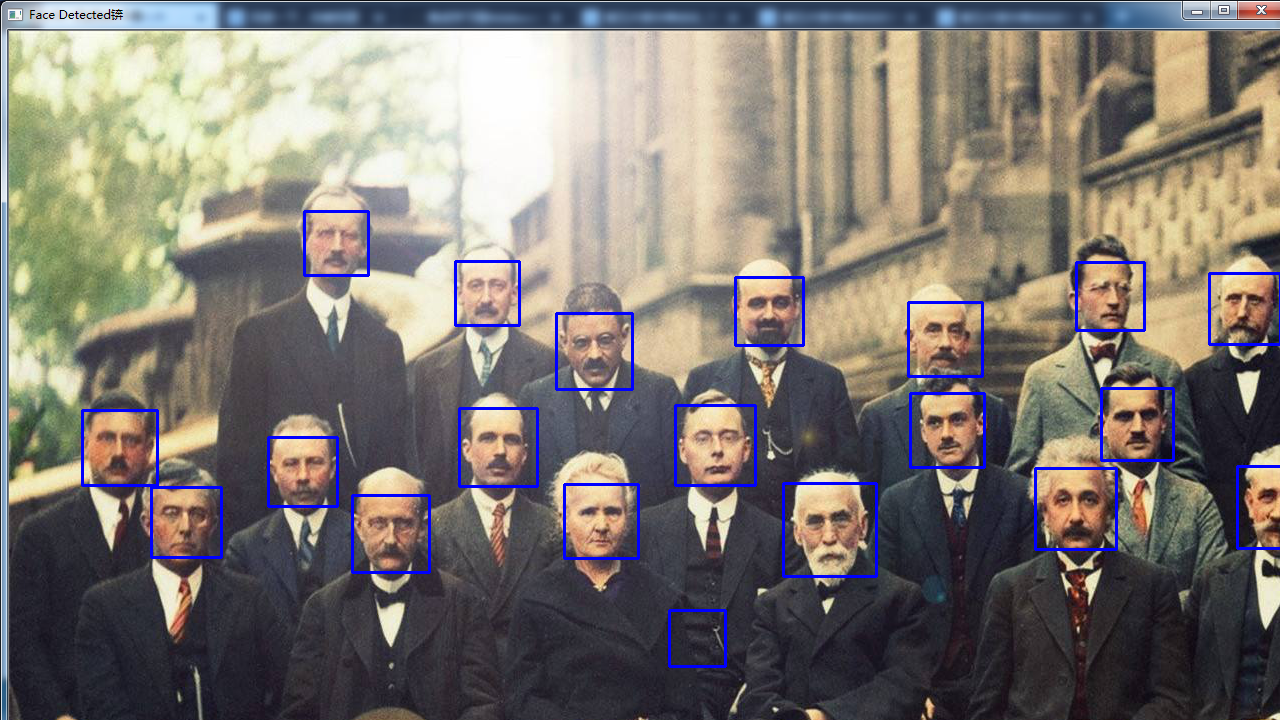#### 2、视频中的人脸检测

#2、视频中的人脸检测
def DynamicDetect():
'''
打开摄像头，读取帧，检测帧中的人脸，扫描检测到的人脸中的眼睛，对人脸绘制蓝色的矩形框，对人眼绘制绿色的矩形框
'''
#创建一个级联分类器 加载一个 .xml 分类器文件. 它既可以是Haar特征也可以是LBP特征的分类器.

#打开摄像头
camera = cv2.VideoCapture(0)
cv2.namedWindow('Dynamic')

while(True):
#读取一帧图像
#判断图片读取成功？
if ret:
gray_img = cv2.cvtColor(frame,cv2.COLOR_BGR2GRAY)
#人脸检测
for (x,y,w,h) in faces:
#在原图像上绘制矩形
cv2.rectangle(frame,(x,y),(x+w,y+h),(255,0,0),2)
roi_gray = gray_img[y:y+h,x:x+w]
#眼睛检测
for (ex,ey,ew,eh) in eyes:
cv2.rectangle(frame,(ex+x,ey+y),(x+ex+ew,y+ey+eh),(0,255,0),2)

cv2.imshow('Dynamic',frame)
#如果按下q键则退出
if cv2.waitKey(100) & 0xff == ord('q') :
break

camera.release()
cv2.destroyAllWindows()

detectMultiScale(image[,scaleFactor[,minNeighbors[,flags[,minSize[,maxSize]]]]])
• image：表示的是要检测的输入图像
• scaleFactor：为每一个图像尺度中的尺度参数，默认值为1.1。scaleFactor参数可以决定两个不同大小的窗口扫描之间有多大的跳跃，这个参数设置的大，则意味着计算会变快，但如果窗口错过了某个大小的人脸，则可能丢失物体。
• minNeighbors：参数为每一个级联矩形应该保留的邻近个数，默认为3。minNeighbors控制着误检测，默认值为3表明至少有3次重叠检测，我们才认为人脸确实存。
• flags：对于新的分类器没有用（但目前的haar分类器都是旧版的，CV_HAAR_DO_CANNY_PRUNING,这个值告诉分类器跳过平滑（无边缘区域）。利用Canny边缘检测器来排除一些边缘很少或者很多的图像区域；CV_HAAR_SCALE_IMAGE，这个值告诉分类器不要缩放分类器。而是缩放图像（处理好内存和缓存的使用问题，这可以提高性能。）就是按比例正常检测；CV_HAAR_FIND_BIGGEST_OBJECTS，告诉分类器只返回最大的目标（这样返回的物体个数只可能是0或1）只检测最大的物，CV_HAAR_DO_ROUGH_SEARCH，他只可与CV_HAAR_FIND_BIGGEST_OBJECTS一起使用，这个标志告诉分类器在任何窗口，只要第一个候选者被发现则结束寻找（当然需要足够的相邻的区域来说明真正找到了。）,只做初略检测.
• minSize：为目标的最小尺寸
• maxSize：为目标的最大尺寸

浅析人脸检测之Haar分类器方法